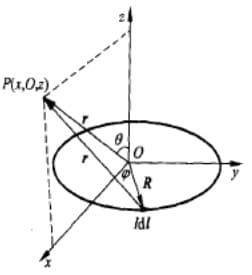# 磁偶极子$p_m=IS$ 称为磁偶极距。

# 磁化

• 抗磁性（水、铜、有机物）：产生的磁场与外加磁场相反（$\mu<\mu_0$）
• 顺磁性（锂、钠、铝、氧气）：产生的磁场与外加磁场相同，磁性较弱（$\mu>\mu_0$）
• 铁磁性（铁、钴、镍、钆）：产生的磁场与外加磁场相同，磁性很强，甚至会大于原有磁场，有磁畴
• 亚铁磁性物质

• 磁介质均匀且介质中无传导电流时，磁化体电流 $J_m=0$（想象内部电流环相互抵消）
• 磁介质表面总有磁化面电流 $J_{ms}\neq 0$
• 穿过整块介质的任意界面上的磁化电流总量等于0，$I_m+I_{sm}=0$（进去的电流等于出去的电流）